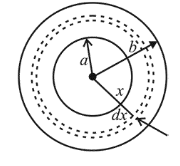# Space between two concentric conducting spheresQuestion:

Space between two concentric conducting spheres of radii $a$ and $b(b>a)$ is filled with a medium of resistivity $p$. The resistance between the two spheres will be :

1. (1) $\frac{p}{4 \pi}\left(\frac{1}{a}-\frac{1}{b}\right)$

2. (2) $\frac{p}{2 \pi}\left(\frac{1}{a}-\frac{1}{b}\right)$

3. (3) $\frac{p}{2 \pi}\left(\frac{1}{a}+\frac{1}{b}\right)$

4. (4) $\frac{p}{4 \pi}\left(\frac{1}{a}+\frac{1}{b}\right)$

Correct Option: 1

Solution:

(1) $d R=\frac{(\rho)(d x)}{4 \pi x^{2}}$

$R=\int d R$

$\int d R=\rho \int_{a}^{b} \frac{d x}{4 \pi x^{2}}$$\Rightarrow R=\frac{\rho}{4 \pi}\left[\frac{-1}{x}\right]_{a}^{b}$

$R=\left(\frac{\rho}{4 \pi}\right) \cdot\left(\frac{1}{a}-\frac{1}{b}\right)$